Scroll

# Useful Keywords for Loops and Table Definitions

Follow
 Summary This article is one in a series of articles that will go through examples of using aggregate script syntax new to Askia 5.3.5.0. Specifically, this article demonstrates how to access properties of loops and the questions within them and also the category index of questions in your rows/cols/edges Applies to Askia Analyse. Written for Researchers, Data Processors, Analysts. Keywords Aggregated script, Calculated variables, Numeric by script, Find all values of a script, Data object, Counts, Parent, Child, Children, FilterByXY, RowSubQuestion, RowQuestion, ColSubQuestion, ColSubQuestion, Nested Edges.

The .qes file and portfolio containing the example discussed is attached: Aggregated Scripts Examples 5.rar.

In this article I will show how to access data in loops from the parent (loop) or the child (question).

Take a look at the Child Counts calculated variable.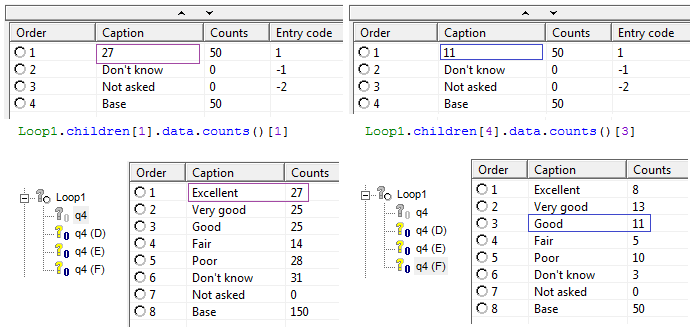• children[n] -  will access the nth child question of the loop
• .data.counts()[n] - will access the counts for the nth code of that question

So above on the left we use this syntax to access the counts for q4 – ‘Excellent’ (27) and above on the right we changed the n values to access the counts for q4 (F) – ‘Good’ (11).

There is also the keyword ‘Parent’ which accesses the data of the loop for a question. E.g. q4.parent.data.counts() will access the counts of the second code of ‘Loop1’.

You can use these keywords in tables by adding them into a calculation arithmetic: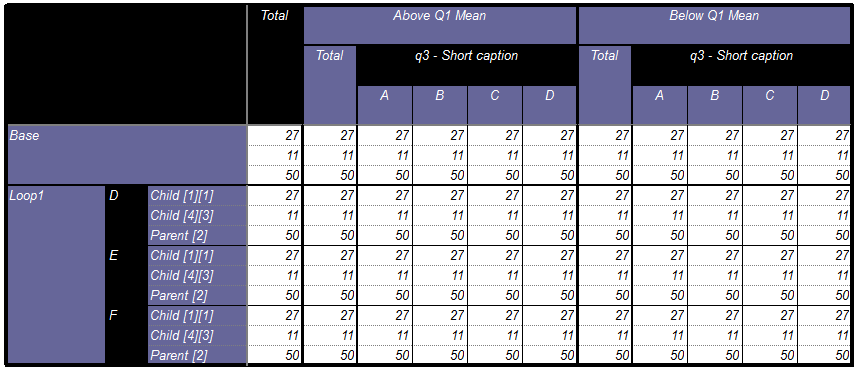In the above case it’s a simple example in which we return the counts to every cell. You can see this by opening up the portfolio in the .qes file provided and viewing the tab definition: (Calc Arithmetic (Parent/Children))

Loop1.children.data.counts()

Loop1.children.data.counts()

q4.parent.data.counts()

However, what will be more useful is if we can return these counts but filter them by the variables and categories in our table.

In this case we amend out scripts to include the FilterByXY keyword (Calc Arithmetic (Parent/Children) - FilterbyXY):

Loop1.children.data.FilterByXY().Counts()

Loop1.children.data.FilterByXY().Counts()

q4.parent.data.FilterByXY().Counts()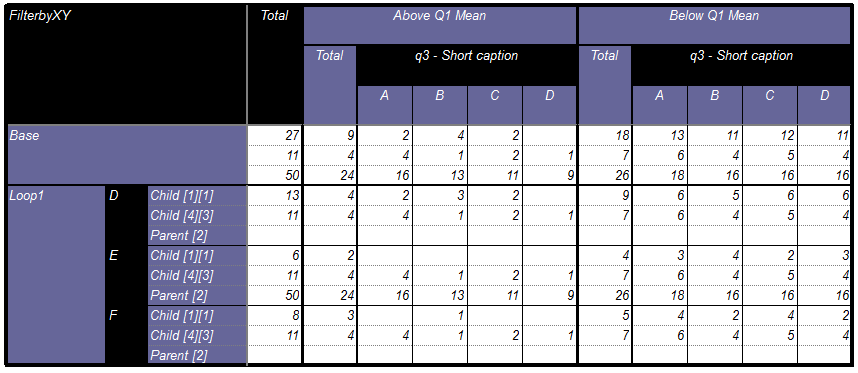As a quick sense check you can see that Parent  doesn’t return anything for the 1st and 3rd iterations of Loop1 because we are only accessing its 2nd code with the syntax.

Imagine you have a loop within a loop, e.g. Loop1, then Loop2, then q4. In this case you can use: q4.parent.parent.data.counts()[n] to access the counts from Loop1.

As a final note on this, you also have the keywords FilterByX and FilterByY.

You may be aware how to use script calculations in Analyse: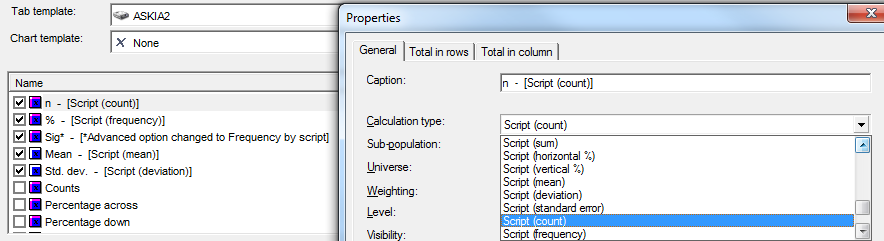If not, there is a fairly informative article here which steps through some usage examples. There is another article here which concentrates on scripts used to create mean summary tables.

Basically, it’s a script stored in a calculation which allows you to access or filter your results by a question which is not in your tab definition.

Open up ‘Aggregated Scripts Examples 5.xml’ and in the tab definition (Script Count (RowSubQuestion)) there is a small example of this in the calculation: ‘n (q4)’. The script here is  ??q4?? Has {1;2}

This filters the counts in our table by those who answered ‘Excellent’ or ‘Very good’ at q4.

This is a question specific script. It means that should we be required to apply this for the same table set-up but different questions then the calculation would not work. e.g. loop1 (replaced with loop2) and q4 (replaced with q5).

In Analyse 5.3.5.0 we have introduced some new keywords to circumvent this inflexibility.

Take a look at the calculation ‘n (Generic)’. The script here is: RowSubQuestion Has {1;2}

The results here are the same as those given by ‘n (q4)’: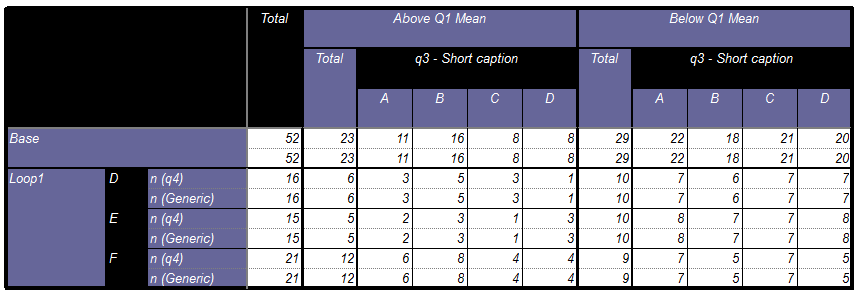The other keywords that can be in these script calculations are:

RowQuestion, ColQuestionColSubQuestion.

Whilst we are looking at keywords for use in you tab definitions, let’s finish off by looking at the Row.Index, Column.Index and Edges[n].Index keywords. Take a look at the Calc Arithmetic (Index) tab definition.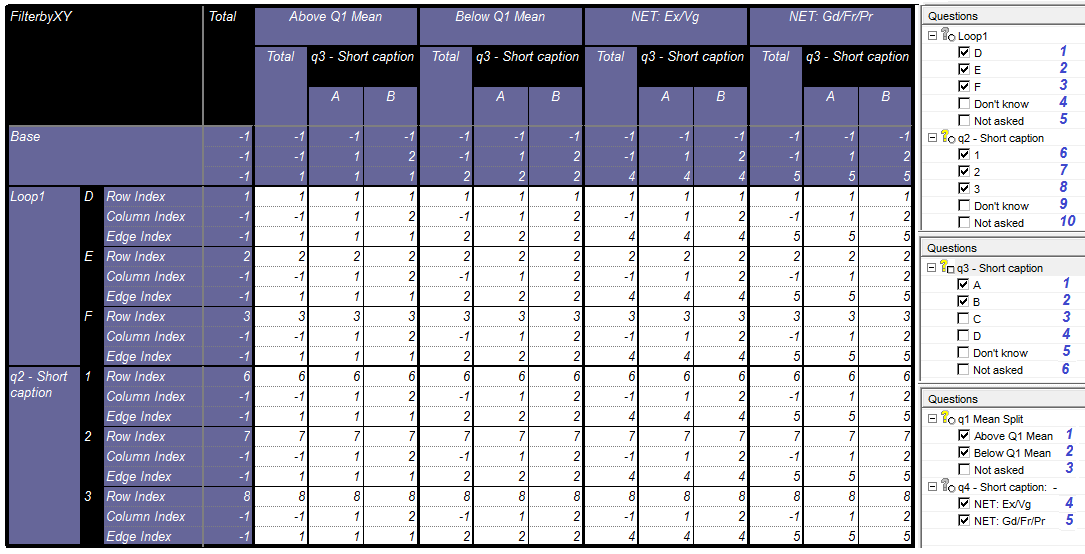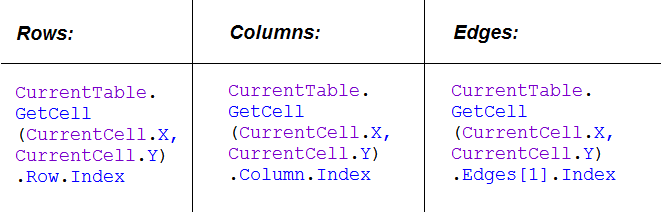Here we make use of Edges. When would we use Edges or Edges?

This is basically when we layer the edge variables upwards rather than sidewards. We call these nested edges. The option can be found in the General tab: Settings > Breaks > Nested edges. More about these here.

 accesses the 2nd variable down in the list of edge variables (in this case, q4 RG) and  would access the 3rd variable of those listed in the edges section of our tab definition.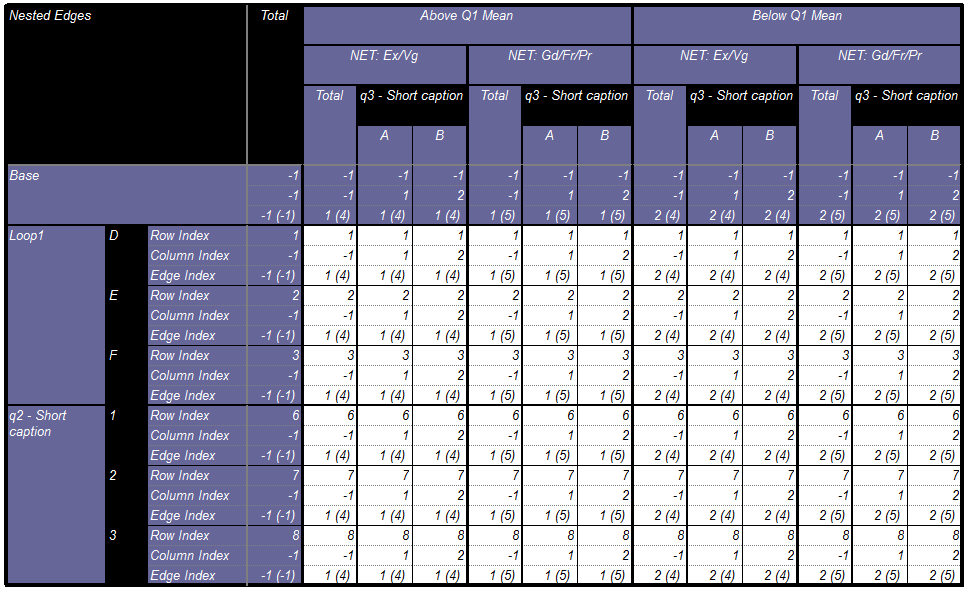In the Edge Index calculation this time I have changed the syntax to be as follows:

CurrentTable.GetCell(CurrentCell.X,CurrentCell.Y).Edges.Index

+"("+

CurrentTable.GetCell(CurrentCell.X,CurrentCell.Y).Edges.Index

+")"

So basically I’m returning the index of the first edge variable followed by the index of 2nd edge variable when nested in brackets.

But look what happens when I turn off the nested edges setting and re-run the table: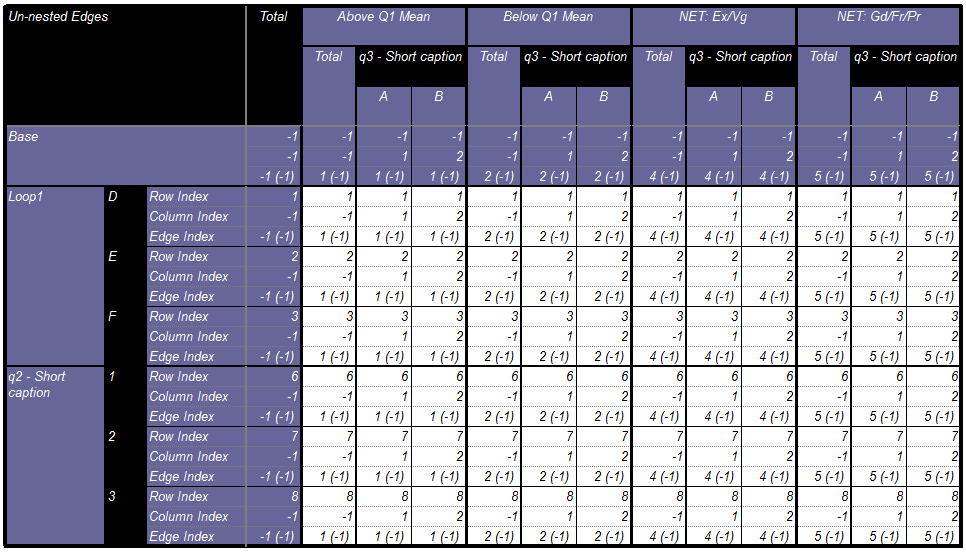Everything that made use of Edges.Index is no longer valid and returns (-1)